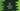# 4 ways to print the Fibonacci series in C### How to print the Fibonacci series in C#:

In this post, we will learn how to print the Fibonacci series in C#. The Fibonacci series or Fibonacci sequence is a series of numbers, which starts with 0 and 1 and each value in this series is equal to the sum of the two preceding numbers.

The starting values of the Fibonacci series are 0, 1, 1, 2, 3, 5, 8, 13, 21….

We can use a simple loop to print this series. The program will take the value of n as input from the user and print the series up to nth term.

Let’s learn how to print this series in different ways.

### Method 1: By using a for loop:

Let’s learn how to use a for loop to print the Fibonacci series in C#:

``````using System;

namespace Program
{
class Program
{
static void Main(string[] args)
{
int n;
int currentValue = 1, prevValue = 0, sum;

Console.WriteLine("Enter the value of n: ");

Console.Write("{0} {1}", prevValue, currentValue);

for (int i = 2; i < n; i++)
{
sum = prevValue + currentValue;
prevValue = currentValue;
currentValue = sum;
Console.Write(" {0}", currentValue);
}
}
}
}``````

Here,

• currentValue is the current value and prevValue is the previous value. The previous value and current values are assigned to 0 and 1 at the beginning of the program.
• These values are printed at the start of the program.
• It uses a for loop to print the final fibonacci series. The loop prints the values from the third number of the series. It adds the previous two values to find the current value of the Fibonacci series. It also updates the prevValue and currentValue on each iteration.

If you run this program, it will print the output as below:

``````Enter the value of n:
10
0 1 1 2 3 5 8 13 21 34``````

### Method 2: By using a separate method and handling edge cases:

The above method will fail for n = 1 or n = 0. It will always print the first two values of the fibonacci series. We can add two more if blocks to handle these cases. Let’s use a separate method to print the series. This method will take the value of n as its argument.

``````using System;

namespace Program
{
class Program
{
static void printFibonacciSeries(int n)
{
if (n == 0) return;

int currentValue = 1, prevValue = 0, sum;

if (n == 1)
{
Console.Write("{0}", prevValue);
return;
}

Console.Write("{0} {1}", prevValue, currentValue);

for (int i = 2; i < n; i++)
{
sum = prevValue + currentValue;
prevValue = currentValue;
currentValue = sum;
Console.Write(" {0}", currentValue);
}
}
static void Main(string[] args)
{
int n;

Console.WriteLine("Enter the value of n: ");

printFibonacciSeries(n);
}
}
}``````

Here,

• printFibonacciSeries method is used to print the Fibonacci series. This method takes the value of n as its parameter.
• If the value of n is equal to 0, it prints nothing and returns.
• If the value of n is equal to 1, it prints only the first value of the series.
• Else, it prints the first two numbers of the series and uses a loop to print other series values.

It will print output as below:

``````Enter the value of n:
0

Enter the value of n:
1
0

Enter the value of n:
10
0 1 1 2 3 5 8 13 21 34``````

### Method 3: By using a while loop:

We can use a while loop to print the Fibonacci series.

``````using System;

namespace Program
{
class Program
{
static void printFibonacciSeries(int n)
{
if (n == 0) return;

int currentValue = 1, prevValue = 0, sum, i = 2;

if (n == 1)
{
Console.Write("{0}", prevValue);
return;
}

Console.Write("{0} {1}", prevValue, currentValue);

while (i < n)
{
sum = prevValue + currentValue;
prevValue = currentValue;
currentValue = sum;
Console.Write(" {0}", currentValue);
i++;
}
}
static void Main(string[] args)
{
int n;

Console.WriteLine("Enter the value of n: ");

printFibonacciSeries(n);
}
}
}``````

It will print similar output.

### Method 4: Recursive way to print the Fibonacci series in C#:

We can also use a recursive method to print the Fibonacci series. A method is called a recursive method if it calls itself again and again to find a result. Let’s write the above program with a recursive method:

``````using System;

namespace Program
{
class Program
{
static void printFibonacciSeries(int first, int second, int n)
{
if (n == 0) return;
Console.Write("{0} ", first);

printFibonacciSeries(second, first + second, n - 1);
}
static void Main(string[] args)
{
int n;

Console.WriteLine("Enter the value of n: ");

printFibonacciSeries(0, 1, n);
}
}
}``````

Here,

• printFibonacciSeries method is a recursive method. It takes three parameters, first, second and n. In the beginning, this method is called with 0 and 1 as first and second. n is the user input value of n.
• If n is equal to 0, it returns. Else it prints the value of first and calls the same method with updated values. The second value is passed as first and first + second is passed as second. Also, the value of n is decremented by 1 on each iteration.

It will handle all edge cases and print output as below:

``````Enter the value of n:
0

Enter the value of n:
1
0

Enter the value of n:
10
0 1 1 2 3 5 8 13 21 34``````